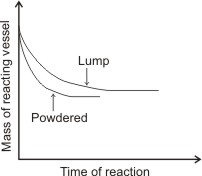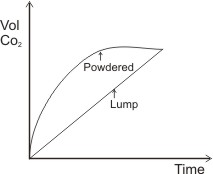# The Rate of a Chemical Reaction

The Rate of a Chemical Reaction
The rate of a chemical reaction can be defined as the amount of the reaction which occurs in unit time. The rate of a reaction is measured by choosing certain properties of the reaction which will indicate how far the reaction has gone, and whose magnitude can be observed with time without disturbing the reaction.

For example, a reaction which shows color change: the rate of such reaction can be measured by observing how the intensity of color formed changes with time. In a similar way, a reaction which involves gases can have its rate measured by collecting the gas and observing the way its volume or mass increases with time. Note: increase in rate of reaction corresponds to decrease in time taken for the reaction, and vice versa.

I.e., rate of reaction is inversely proportional to time,

R α1/T

Factors which affect the Rate of Chemical Reactions

Factors which can affect the rate of a chemical reaction include:

1. Temperature

By the kinetic theory, chemical reactions occur due to collisions of molecules, atoms or ions involved in the reaction. When the temperature of a reacting system is increased by way of heating, the reacting particles gain energy and are able to move faster, resulting in increased collisions, which increases the rate of the reaction.

An illustration of this can be found in the reaction between sodium trioxo sulphur sulphate(VI) solution and hydrochloric acid. The reaction leads to the precipitation of sulphur.

By observing the precipitation of sulphur at various temperatures with time, the result obtainable would indicate that: as the temperature of the reaction is increased, the time taken to precipitate sulphur is decreased (i.e. rate of reaction increases).

Note: the way by which the intensity of the precipitate can be measured is to carry out the entire reaction in a beaker, and by placing the beaker and its contents on a white piece of paper with a cross marked on it. The time for the disappearance of the cross when the contents of the beaker are viewed from above will give a measure of the time taken for a certain fraction of the reaction to occur (i.e. the rate of the reaction) – a stop clock or a watch is used to note this time.

By plotting the graph of temperature over time of reaction, we obtain the curve:Note: the curve slopes from left to right indicating that increase in temperature leads to decrease in time (i.e., increase in rate of reaction) and vice versa. Thus, temperature is inversely proportional to time (Temp α 1/Time).

In general, increase in temperature of a reaction by 100 results in doubly the rate of reaction (i.e. decrease in time taken by half).

1. Concentration of Reactants

The concentration or density of reacting substances affect their closeness to one another, and consequently the frequency of their collision – this ultimately affects the rate of reaction. The higher the concentration of reactants, the greater the rate of reaction and vice-versa.

To investigate the effect of concentration on the rate of reaction, the reaction between dilute HCl and sodium trioxo sulphur sulphate (VI) solution to precipitate sulphur may be studied.

By observing the reactions of different concentrations of sodium trioxo sulphur sulphate(VI) with fixed concentration of HCl, it would be observed, from the result of the reaction that the higher the concentration, the shorter will be the time taken for sulphur to precipitate, i.e., the higher the concentration, the greater the rate of reaction.

To observe the intensity of sulphur produced at any given moment (this represents the extent of the reaction, i.e. the rate of the reaction), the reaction is carried out in a beaker, placed on a piece of paper with a cross marked on it. The time taken for the cross to be invisible when the content of the beaker is viewed from above is noted using a stop clock or a watch – this is the time taken for a certain fraction of the reaction to occur.

By plotting the graph of concentration against time of reaction, the following curve is obtained:The curve indicates concentration varying inversely with time, i.e., conc. α 1/T. Hence, increasing the concentration of reacting masses leads to decrease in time of reaction (i.e. increase in rate of reaction). Decreasing the concentration of reacting masses leads to increase in the time of reaction (i.e. decrease in rate of reaction).

Another study to show the effect of concentration of reactants on the rate of reaction is “the iodine-clock reaction” – this is the reaction between hydrogen peroxide solution and acidified potassium iodide solution. Iodine is produced and is indicated by the observation of a dark-blue coloration with starch.

2I(aq),+ 2H+(aq) + H2O2(aq) → 2H2O (l) + I2(aq)

The procedure is to use a fixed concentration of one reactant together with a variable concentration of the other, and to observe the rate of reaction in each combination.

The result of the study would show that the rate of reaction increases with increase in concentration of the reactants. For gaseous reactions, increase of pressure implies increase of concentration – the rate of the reaction tends to increase as a result of increase in collisions.

Note: generally, increase in the concentration (in moles per dm3 or mmHg for gases) of reactants will result in increase in rate of reaction – i.e. decrease in reaction time – and vice versa. The graph above applies to all similar cases.

1. Surface Area of Reactants

In heterogeneous reactions – reactions in which all the reactants are not in the same phase (i.e. different states) – the surface area of the reactants have been found to affect the rate of the reactions. For example, comparing the rate of reactions of:

(i) Dilute HCl and marble chips.

(ii) Dilute HCl and powdered marble of the same mass as

(i). CaCO3(s) + 2HCl(aq → CaCl2(aq) + CO2(s) + H2O

(l) Both reactions produce CO2 gas. This causes a reduction in the mass of the reacting vessel with time, and this may be taken as a measure of the rate of the reaction.

The result of both reactions would show that the rate of reaction of the powdered marble is greater than that of the chip (as shown in the graph below – the mass of the reacting vessel of the powdered marble reduces at shorter time than the mass of the reacting vessel of the lump of marble).

The reason for this is that the surface area of the powdered marble, being very large compared with that of the chip is responsible for the difference in their reaction rates.

The graph of the mass of reacting vessel against time of reaction is similar to the diagram below:

Note: The two curves level off at the same point. The reason for this is that the chip (or large piece) completely dissolves after some time, then the rate of its reaction becomes equal to that of the powdered one.

In general, for heterogeneous reactions, reactants of large surface areas have greater rates of reaction (i.e., reactions occur at shorter time) than reactants of small surface areas. The surface area of powder is greater than that of lumps or large piece.

Another way the rates of the above reactions can be measured is to measure their increasing volume of CO2 produced with time. The graph of volume of CO2 produced against time will give:

1. The Use of Catalyst

The presence of catalysts in chemical reactions would increase their rate.

UKPCS Notes brings Prelims and Mains programs for UKPCS Prelims and UKPCS Mains Exam preparation. Various Programs initiated by UKPCS Notes are as follows:- For any doubt, Just leave us a Chat or Fill us a querry––
error: Content is protected !!